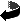# Algebras of pseudodifferential operators on complete manifolds by Bernd Ammann, Robert Lauter, Victor Nistor

Algebras of pseudodifferential operators on complete manifolds (.dvi, .ps,.ps.gz oder .pdf)
Electronic Research Announcements of the AMS 9 (2003), 80-87.

### Abstract

Melrose has studied examples of non-compact manifolds $M_0$ whose large scale geometry is described by a Lie algebra of vector fields $\VV \subset\Gamma(M;TM)$ on a {\em compactification} of $M_0$ to a manifold with corners $M$. The geometry of these manifolds -- called manifolds with a Lie structure at infinity'' -- was studied from an axiomatic point of view in \cite{aln1}. In this paper, we define and study an algebra $\Psi_{1,0,\VV}^\infty(M_0)$ of pseudodifferential operators canonically associated to a manifold $M_0$ with a Lie structure at infinity $\VV \subset \Gamma(M;TM)$. We show that many of the properties of the usual algebra of pseudodifferential operators on a compact manifold extend to the algebras that we introduce. In particular, the algebra $\Psi_{1,0,\VV}^\infty(M_0)$ is a microlocalization'' of the algebra $\DiffV{*}(M)$ of differential operators with smooth coefficients on $M$ generated by $\VV$ and $\CI(M)$. This proves a conjecture from \cite{meicm}.

### Keywords

Keywords!!!!!Zurück zur Homepage

Bernd Ammann, Robert Lauter, Victor Nistor,
The Paper was written on 19.03.2003
Last update 10.7.2003